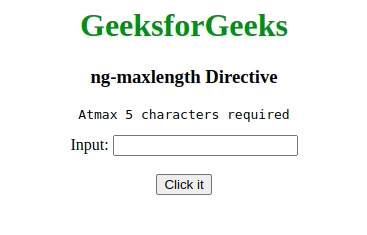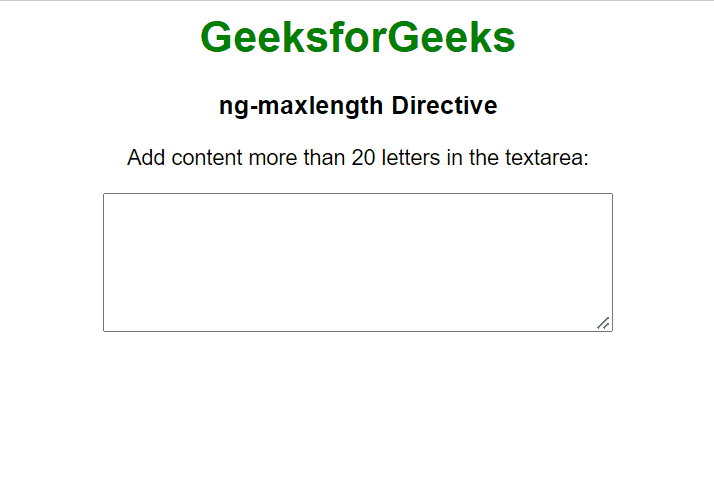Open in App
Not now

# AngularJS ng-maxlength Directive

• Last Updated : 06 Sep, 2022

The ng-maxlength Directive in AngularJS is used to set the maximum length for an input field i.e it adds the restriction for an input field. It is different from maxlength attribute in HTML because the former prevents users from exceeding the limit whereas the later doesn’t do that. It will make the form invalid if the input limit exceeds it.

Syntax:

```<element ng-maxlength="expression">
Contents...
</element> ```

Parameter value:

• expression: This is a number denoting the maximum limit up to which the input is valid.

Example: This example uses the ng-maxlength Directive to check the maximum length of the string.

## HTML

 ```<``html``>`` ` `<``head``>``    ``<``title``>ng-maxlength Directive``    ``<``script` `src``=``"https://ajax.googleapis.com/ajax/libs/angularjs/1.6.9/angular.min.js"``>``    `````` ` `<``body` `style``=``"text-align: center"``>``    ``<``h1` `style``=``"color:green"``>GeeksforGeeks``    ``<``h3``>ng-maxlength Directive``    ``<``div` `ng-app``=``"app"` `         ``ng-controller``=``"geek"``>``        ``<``form` `name``=``"maxlen"` `              ``novalidate ``ng-submit``=``"maxlen.\$valid &&maxlength()"``>``            ``<``pre``>Atmax 5 characters required``            ``Input: ``            ``<``input` `type``=``"text"` `                   ``name``=``"code"` `                   ``ng-model``=``"txt"` `                   ``ng-maxlength``=``"5"` `required />``            ``<``br``><``br``>``            ``<``button` `type``=``"submit"``>Click it``            ``<``br``><``br``>``            ``<``span``>{{msg}}``        ````    ```` ` `    ``<``script``>``        ``var app = angular.module('app', []);``        ``app.controller('geek', function (\$scope) {``            ``\$scope.maxlength = function () {``                ``\$scope.msg = "Input is valid";``            ``};``        ``});``    ```````

Output:Example 2: This example describes the usage of the ng-maxlength Directive in AngularJS by displaying the error message if the length of the text exceed from 20 letters.

## HTML

 ```<``html``>`` ` `<``head``>``    ``<``script` `src``=``"https://ajax.googleapis.com/ajax/libs/angularjs/1.6.9/angular.min.js"``>``    ````    ``<``style``>``        ``body {``            ``text-align: center;``            ``font-family: Arial, Helvetica, sans-serif;``        ``}`` ` `        ``h1 {``            ``color: green;``        ``}`` ` `        ``h4 {``            ``font-size: 25px;``            ``color: rgb(239, 58, 58);``        ``}``    `````` ` `<``body` `ng-app``=``""``>``    ``<``h1``>GeeksforGeeks``    ``<``h3``>ng-maxlength Directive``    ``<``form` `name``=``"gfgFormData"``>``        ``<``p``>``            ``Add content more than 20``            ``letters in the textarea:``        ````        ``<``textarea` `type``=``"textarea"` `                  ``name``=``"geeks"``                  ``ng-model``=``"geeks"` `                  ``ng-maxlength``=``"20"``>``        ````        ``<``h4` `ng-if``=``"!gfgFormData.geeks.\$valid"``>``            ``Entered text length is more than 20 letters``        ````    ```````

Output:My Personal Notes arrow_drop_up